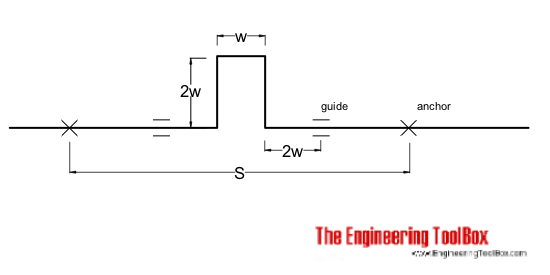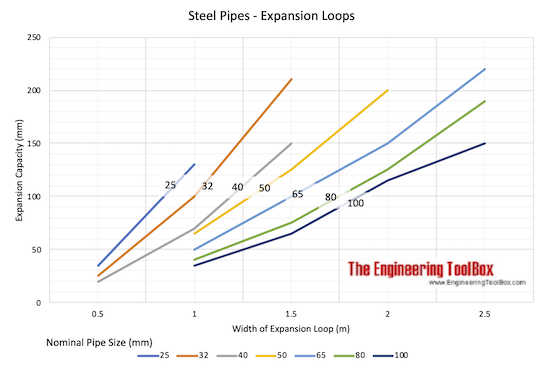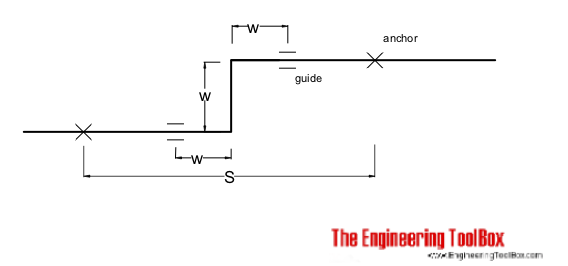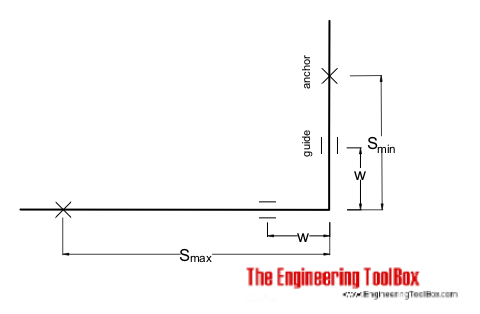Engineering ToolBox - Resources, Tools and Basic Information for Engineering and Design of Technical Applications!

# Steel Pipes - Calculate Thermal Expansion Loops

## Calculating and sizing steel pipe thermal expansion loops.

The expansion loop is a common way to absorb temperature expansion and contraction in steel pipes. Expansion loops can be fabricated from standard pipes and elbows.Approximate Expansion Capacity (mm)
Nominal Pipe Size
(mm)
Width of Expansion Loop - w - (m)
0.51.01.52.02.5
25 35 130
32 25 100 210
40 20 70 150
50   65 125 200
65   50 100 150 220
80   40 75 125 190
100   35 65 115 150### U-Bends Expansion Compensator Calculator

#### Metric Units

This calculator can be used to calculate required width and height of an U-bends expansions compensator.

S - Length of expanding pipe (m)

Expansion coefficient (10-6 m/m oC)

Initial temperature (oC)

Final temperature (oC)

Outside diameter of pipe (mm)

#### Imperial Units

S - Length of expanding pipe (ft)

Expansion coefficient (10-6 in/in oF)

Initial temperature (oF)

Final temperature (oF)

Outside diameter of pipe (in)

The U-loops calculation is based on equation

w = 0.015 (dl D)1/2       (1)

where

w = width of loop (m)

dl = α dt S = temperature expansion (m)

α = temperature expansion coefficient (m/m oC)

dt = temperature change (oC)

S = length of expanding pipe (m)

D = diameter of pipe (m)

The offset of the loop is 2 w. The length of the loop is 5 w.

### Z-Bends Expansion Compensator Calculator#### Metric Units

This calculator can be used to calculate required offset in a Z-bends expansions compensator.

S - Length of expanding pipe (m)

Expansion coefficient (10-6 m/m oC)

Initial temperature (oC)

Final temperature (oC)

Outside diameter of pipe (mm)

#### Imperial Units

S - Length of expanding pipe (ft)

Expansion coefficient (10-6 in/in oF)

Initial temperature (oF)

Final temperature (oF)

Outside diameter of pipe (in)

### L-Bends Expansion Compensator Calculator#### Metric Units

This calculator can be used to calculate required offset in a Z-bends expansions compensator.

Smax - Length of longest expanding leg (m)

Expansion coefficient (10-6 m/m oC)

Initial temperature (oC)

Final temperature (oC)

Outside diameter of pipe (mm)

#### Imperial Units

This calculator can be used to calculate required offset in a Z-bends expansions compensator.

Smax - Length of longest expanding leg (ft)

Expansion coefficient (10-6 in/in oF)

Initial temperature (oF)

Final temperature (oF)

Outside diameter of pipe (in)

The L-loop calculation is based on equation

L = 0.063 (dl D)1/2       (2)

where

L = length of loop (m)

## Related Topics

• Temperature Expansion - Thermal expansion of pipes and tubes - stainless steel, carbon steel, copper, plastics and more.
• Pipe Sizing - Sizing of steam and condensate pipe lines - pressure loss, recommended velocity, capacity and more.

## Engineering ToolBox - SketchUp Extension - Online 3D modeling!

Add standard and customized parametric components - like flange beams, lumbers, piping, stairs and more - to your Sketchup model with the Engineering ToolBox - SketchUp Extension - enabled for use with the amazing, fun and free SketchUp Make and SketchUp Pro .Add the Engineering ToolBox extension to your SketchUp from the SketchUp Pro Sketchup Extension Warehouse!

Translate

## Privacy

We don't collect information from our users. Only emails and answers are saved in our archive. Cookies are only used in the browser to improve user experience.

Some of our calculators and applications let you save application data to your local computer. These applications will - due to browser restrictions - send data between your browser and our server. We don't save this data.

## Citation

• Engineering ToolBox, (2008). Steel Pipes - Calculate Thermal Expansion Loops. [online] Available at: https://www.engineeringtoolbox.com/steel-pipe-expansion-loop-d_1069.html [Accessed Day Mo. Year].

Modify access date.

. .

#### Scientific Online Calculator6 24